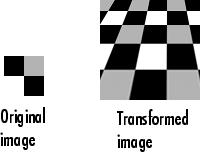## N-Dimensional Spatial Transformations

You can perform N-D geometric transformations using the `tformarray` function. You can also use `tformarray` to perform mixed-dimensional transformations, in which the input and output arrays do not have the same dimensions. The output can have either a lower or higher number of dimensions than the input. For example, if you are sampling 3-D data on a 2-D slice or manifold, the input array might have a lower dimensionality. The output dimensionality might be higher, for example, if you combine multiple 2-D transformations into a single 2-D to 3-D operation.

Before using the `tformarray` function, prepare the input arguments required to perform the geometric transformation.

• Create the spatial transformation using the `maketform` function. If you create the spatial transformation from a matrix, `maketform` expects the matrix to be in the postmultiply convention.

• Create the resampling structure using the `makeresampler` function. A resampler structure defines how to interpolate values of the input array at specified locations. For example, you could specify your own separable interpolation kernel, build a custom resampler around the `interp2` or `interp3` functions, or even implement an advanced antialiasing technique. The resampling structure also controls the edge behavior when interpolating.

Next, apply the geometric transformation to an image using the `tformarray` function, specifying the spatial transformation structure and the resampling structure. You can also transform individual points and lines to explore the geometric effects of a transformation. Use the `tformfwd` and `tforminv` functions to perform forward and inverse transformations, respectively.

This example uses `tformarray` to perform a projective transformation of a checkerboard image, and `makeresampler` to create a resampling structure with a standard bicubic interpolation method.

```I = checkerboard(20,1,1); figure imshow(I) T = maketform("projective",[1 1; 41 1; 41 41; 1 41],... [5 5; 40 5; 35 30; -10 30]); R = makeresampler("cubic","circular"); J = tformarray(I,T,R,[1 2],[2 1],[100 100],[],[]); figure imshow(J)```The `makeresampler` and `tformarray` functions enable you to control many aspects of the transformation. For example, note how `tformarray` created an output image that is larger than the size of the original image. Further, notice that the transformed image appears to contain multiple copies of the original image. This is accomplished by specifying a padding method in the resampling structure that extends the input image by repeating the pixels in a circular pattern.